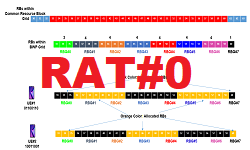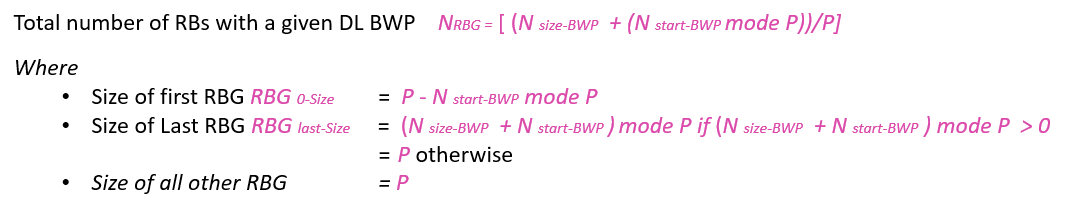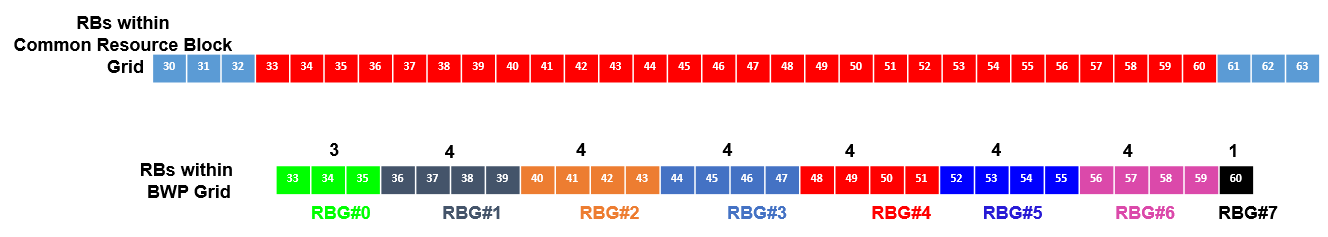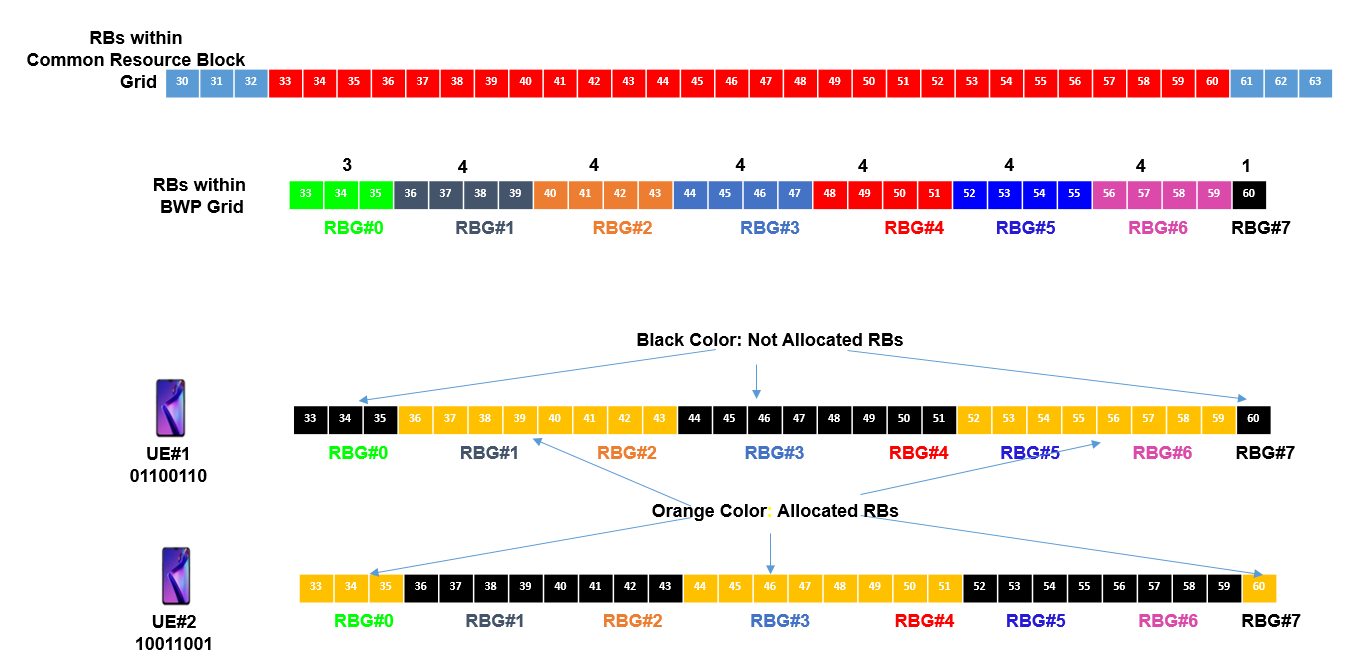# 5G NR Resource Allocation – Resource Allocation Type 0

Introduction

To transmit and recieved data in downlink and uplink UEs need bandwidth resource allocated and this allocation provided by gNB. In 5G NR, similar to 4G the resource allocation is done in Time domain and frequency domain. Time domain resource allocation defines which symbols are allocated to UE and frequency domain allocation defines which RBs are allocated to the UE.

3GPP specification has defines following two type of resource allocation types in frequency domain:

• Resource Allocation Type #0 (RAT#0)
• Resource Allocation Type #1 (RAT#1)

In this post we will discuss about RAT#0 frequency domain allocation.

Key Pointers

• DL Resource Allocation Type 0 (RAT #0) can be signalled on the PDCCH using DCI Format 1_ 1
•  RAT #0 resourse allocation use non-interleaved VRB-to-PRB mapping i.e. VRBn is directly mapped to PRBn
• RAT #0 resource allocation uses a bitmap to indicate a set of allocated Resource Block Groups (RBG)
• Resource Block Group defines a set of RBs and each RBG is denoted by a single bit
• RBG bitmap is such that RBG#0 to RBG#NRBG−1 are mapped from MSB to LSB of the bitmap
• The no. of RBs within a RBG is provided by RRC parameter ‘rbg-Size’ within PDSCH-config
• NR resource allocation support two configurations config#1 and config#2
• NR frequency resources are allocated per BWP

How UEs knows which Resource Allocation Type is used by gNB

The gNB informs the UE about which resource allocation scheme to be used via RRC signalling within PDSCH-Config following parameter

• resourceAllocation          ENUMERATED  {resourceAllocationType0, resourceAllocationType1, dynamicSwitch}
• resourceAllocationType0: RAT#0 should be used.
• resourceAllocationType1: RAT#1 should be used.
• dynamicSwitch: RAT selection is left flexible, which means either type 0 or type 1 shall be used as indicated by DCI. The MSB bit of the field Frequency domain resource assignment within the DCI 1_1/1_2 is used to indicate resource allocation type 0 or type 1 if bit value = 0 use RAT#0 and if bit value = 1 use RAT#1.

Relationship with Bandwidth Part (bwp), RBG config and rbg-Size

Following table shows the relationship between btw, rbg-config and rbg-Size. We have considered a total channel bandwidht having total resource block 275 with four bandwidth parts. config#1 minimum rbg-Size is 2 RBs and maximum it can be 16 RBs where as config#2 minimum rbg-Size is 4 RBs and maximum it can be 16 RBs. These rbg-Size associated with the respective bandwidth part shown in following table.Calculating the RBs with in different RBG

While allocating the resource blocks (RBs) within different RBG, it is not neccessarly tha all the RBGs will have equal no. of RBs, the First and the Last RBGs in can have less number of RBs than specified for that particulator BWP. The bitmap is of size NRBG and varies depending upon the size of the bandwidth part and the position of the bandwidth part within the Common Resource Block (CRB) grid, and RBG size (P). Following formula can be used to calculated.Case #1:  RB value that is an integer multiple of RBG size and RBG with BWP have equal no. of RBs

• Start RB of BWP = Nstart-BWP  = 32
• Bandwidht Part Size = Nsize-BWP  = 24
• rbg config = config#2
• rbg-Size from table = P = 4
• Total no. of bits of NRBZ  = 6
• Size of  First RBS = RBG0-Size  = 4
• Size of Last RBG = RBGlast-Size = 4
• Size of other RBG = P = 4Case #2:  RB value that is an integer multiple of RBG size and RBG at lower edge BWP have less no. of RBs

• Start RB of BWP = Nstart-BWP  = 33
• Bandwidht Part Size = Nsize-BWP  = 23
• rbg config = config#2
• rbg-Size from table = P = 4
• Total no. of bits of NRBZ  = 6
• Size of  First RBS = RBG0-Size  = 3
• Size of Last RBG = RBGlast-Size = 4
• Size of other RBG = P = 4Case #3: RB value that is not an integer multiple of RBG size and RBG at upper and lower edge of BWP have less no. of RBs

• Start RB of BWP = Nstart-BWP  = 33
• Bandwidht Part Size = Nsize-BWP  = 28
• rbg config = config#2
• rbg-Size from table = P = 4
• Total no. of bits of NRBZ  = 8
• Size of  First RBS = RBG0-Size  = 3
• Size of Last RBG = RBGlast-Size = 1
• Size of other RBG = P = 4Resource Block Allocation Example

Lets consider UE#1 and UE#2.  UE1’s resource allocation bitmap = 01100110   and UE2’s bitmap = 10011001. If the bit value is ‘1’ then it can be considered that RBG is allocated to UE and if bit value is ‘0’ then RBG is not allocated. This bitmap for RBG allocation it provided via DCI to the UEs. The following figure illustrates which RBs are allocated to UE1 and UE2.

• Start RB of BWP = Nstart-BWP  = 33
• Bandwidht Part Size = Nsize-BWP  = 28
• rbg config = config#2
• rbg-Size from table = P = 4
• Total no. of bits of NRBZ  = 8
• Size of  First RBS = RBG0-Size  = 3
• Size of Last RBG = RBGlast-Size = 1
• Size of other RBG = P = 4
• UE1’s bitmap = 01100110
• UE2’s bitmap = 10011001References:

• 3GPP TS 38.211  5G NR, Physical channels and modulation
• 3GPP TS 38.212 5G NR, Multiplexing and channel coding.
• 3GPP TS 38.214 5G NR, Physical layer procedures for data
• 3GPP TS 38.331 5G  NR, Radio Resource Control (RRC)

Related Topics: